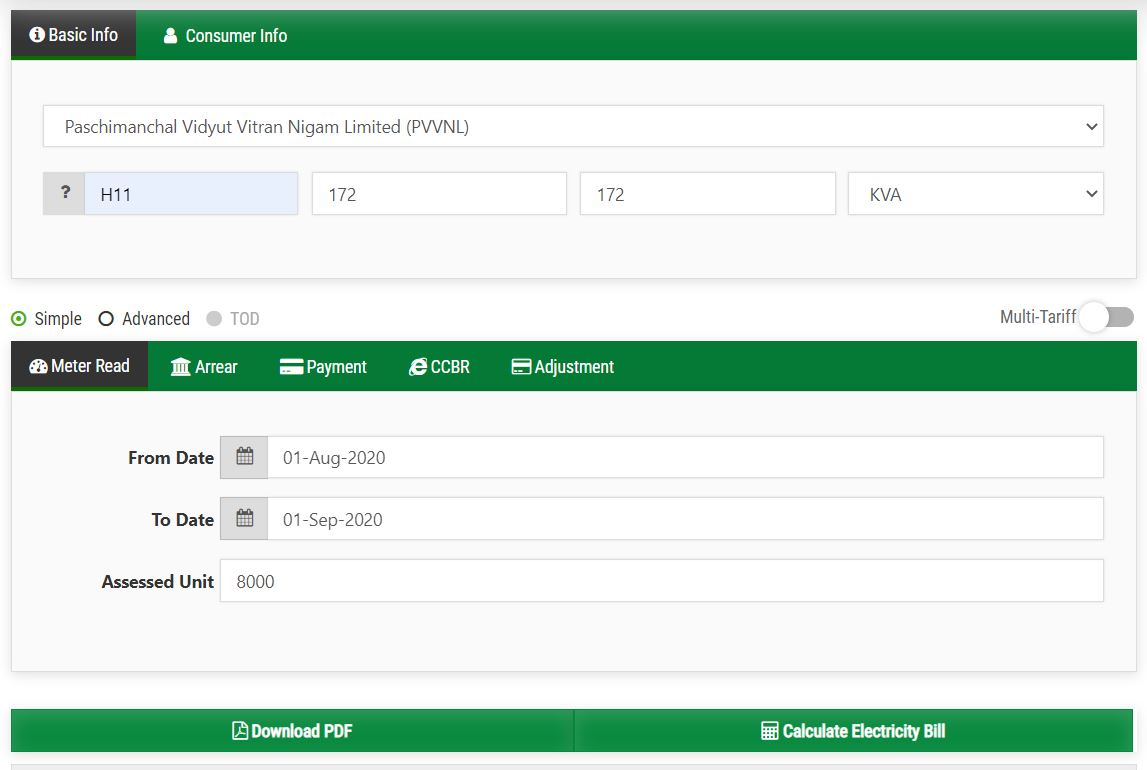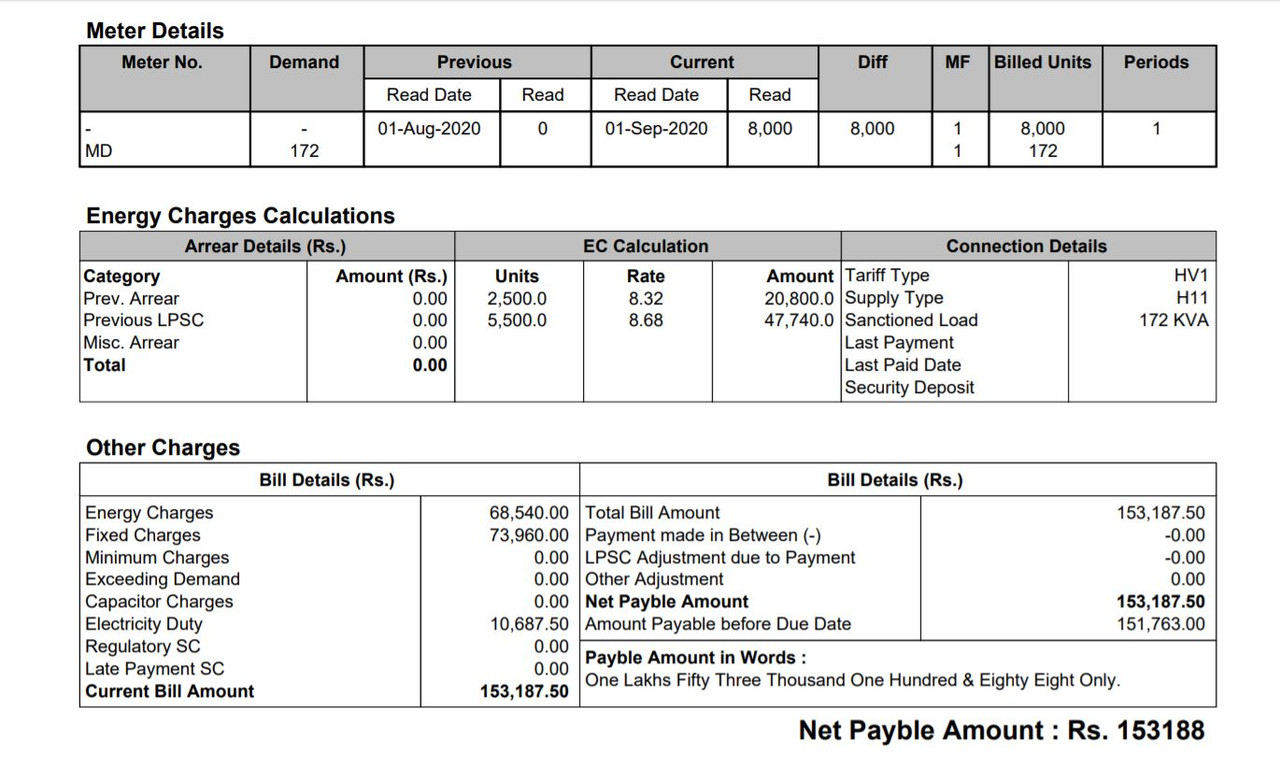#### kw into KVAMember ·  2

Hello i am a bulk consumer having load of 675kw. Supply type is HV1 and H11.
Need to know following
1) how is Kw converted into KVA?
2) fixed charges are billed for full load converted into KVA or some percent of full load converted into KVA?

Last edited by Rahulraghav (28 July 2020 17:23)Tariff rate is being converted into KVAH based with multiplying factor of 0.9

KW = KVA x power factor.

UPPCL has assumed power factor as 0.9 for tariff conversion between KW and KVA

If your billing is KVAH based.
Electricity charges will be converted as follows:
1. Electricity rate will be 0.9 x KWH rate. ( Rs. 5.50/KWH = Rs. 4.95/KVAH)
2. Unit slab will also be changed with MF = 1/0.9 (1st slab is 150 KWH = 167 KVAH)
3. Fixed charges will be changed with MF 0.9 (Rs. 110/KW = Rs. 99/KVA)

Fixed charges is calculated as follows:
1. if Max demand < 75% of sanctioned load, then 75% of sanctioned load
2. if Max demand is greater than 75% and less than 100% of sanctioned load, then on max demand
3. if Max demand > 100% of sanctioned load, then penalty will be imposedMember ·  2

Thank you sir for your reply. I have understood how the fixed charges are calculated vis a vis demand/load and power factor assumption. Please make me understand the folllowing.
I have taken 675 kw connection and further have given sub connection of 155kw to my tenant. The tenant pays as per uppcl guidelines which is Rs 435 per kva. Assuming that he is using 100percent of his sanctioned load, what shall be the following :-
1) tenant's connection load in kva? Is it 155÷0.90?
2) fixed charges in rupees?
2) unit charges? Is it 8.68 per unit?

Last edited by Rahulraghav (2 August 2020 16:38)1. Sanctioned load = 172 KVA
2. Fixed Charges = Rs. 430/KVA = Rs. 73960 (assuming, he is using 100% load and you are not giving him benefit of less MD)
3. Rs. 8.32 for first 2500 units and rest units at Rs. 8.68/units. (Here units will be KVAH, not KWH)